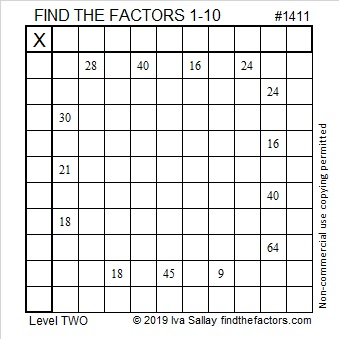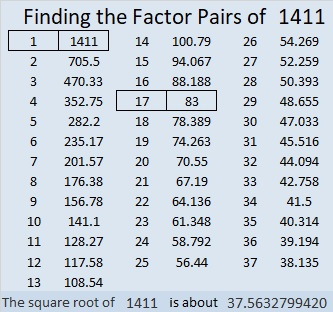# 1411 and Level 2

Four of the fourteen clues, 18, 24, 16, and 40, appear twice in this puzzle, but do they lead you to the same factors? Where do the factors from 1 to 10 belong that will make this puzzle function like a multiplication table?Print the puzzles or type the solution in this excel file: 10 Factors 1410-1418

Now I’ll write a little bit about the puzzle number, 1411:

• 1411 is a composite number.
• Prime factorization: 1411 = 17 × 83.
• 1411 has no exponents greater than 1 in its prime factorization, so √1411 cannot be simplified.
• The exponents in the prime factorization are 1, and 1. Adding one to each exponent and multiplying we get (1 + 1)(1 + 1) = 2 × 2 = 4. Therefore 1411 has exactly 4 factors.
• The factors of 1411 are outlined with their factor pair partners in the graphic below.1411 is the hypotenuse of a Pythagorean triple:
664-1245-1411 which is (8-15-17) times 83.

This site uses Akismet to reduce spam. Learn how your comment data is processed.